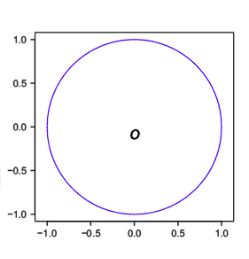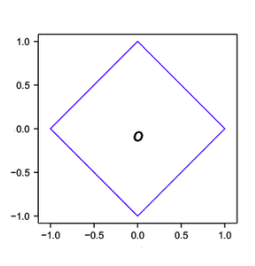Similarity measures and distances: Basic reference and examples for data science practitioners

# Similarity measures and distances: Basic reference and examples for data science practitioners

Last updated:

WIP Alert This is a work in progress. Current information is correct but more content may be added in the future.

## Euclidean distance

$$\text{dist}(\vec{x},\vec{y})=\sqrt{\sum^n_{i=1} (x_i - y_i)^2}$$

• When to use

• When you have normalized vectors.All points in the blue circle have the same Euclidean distance to the origin.

## Manhattan distance

Also called "city-block" distance

$$\text{dist}(\vec{x},\vec{y})=\sum^n_{i=1} |x_i - y_i|$$All points in the blue square have the same Manhattan distance to the origin.

## Mahalanobis distance

$$\text{dist}(\vec{x},\vec{y})= \sqrt{(\vec{x} - \vec{y})' {\rm \bf C}^{-1} (\vec{x} - \vec{y}) }$$

where $$\vec{x}$$ and $$\vec{y}$$ are two points from the same distribution which has covariance matrix $${\rm \bf C}$$.

The Mahalanobis distance takes into account how spread apart points are in the dataset (i.e. the variance of the dataset) to weigh the absolute distance from one point to another.For a very skewed dataset (such as the one shown on this picture),
the Mahalanobis distance (right) may be a better and more
intuitive distance metric.
Source: UWaterloo Stats Wiki

Intuitively: an absolute distance of 2 in a dataset that's very compact (i.e. low-variance) is more significant than an absolute distance of 2 in a dataset where points are normally very much apart from each other (i.e. high variance).

• When to use

• When your points are correlated
• When the covariance of the points is very far from spherical.
• Notes

• The Mahalanobis distance corresponds to the Euclidean distance when the points are uncorrelated (i.e. when the covariance matrix is the identity matrix)

## Cosine distance

$$\text{dist}(\vec{x},\vec{y})=\frac{\vec{x} \bullet \vec{y}}{ \sqrt{\vec{x} \bullet \vec{x}} \sqrt{\vec{y} \bullet \vec{y}}}$$

where $$\bullet$$ is the dot product between $$\vec{x}$$ and $$\vec{y}$$.

Cosine similarity measures the angle between two vectors in the vector space.

• When to use

• When you do not want to take vector magnitudes into account, only their directions (for example, when using word frequencies to represent a document).
• Notes

• Cosine distance is the same for normalized and unnormalized vectors.